Courses

# Flanged Beams - Numerical Problems (Part - 2) Civil Engineering (CE) Notes | EduRev

## Civil Engineering (CE) : Flanged Beams - Numerical Problems (Part - 2) Civil Engineering (CE) Notes | EduRev

The document Flanged Beams - Numerical Problems (Part - 2) Civil Engineering (CE) Notes | EduRev is a part of the Civil Engineering (CE) Course RCC & Prestressed Concrete.
All you need of Civil Engineering (CE) at this link: Civil Engineering (CE)

Instructional Objectives: At the end of this lesson, the student should be able to:

• identify the two types of problems – analysis and design types,
• apply the formulations to design the flanged beams.

5.12.1 Introduction

Lesson 10 illustrates the governing equations of flanged beams and Lesson 11 explains their applications for the solution of analysis type of numerical problems. It is now necessary to apply them for the solution of design type, the second type of the numerical problems. This lesson mentions the different steps of the solution and solves several numerical examples to explain their step-by-step solutions.

5.12.2 Design Type of Problems

We need to assume some preliminary dimensions of width and depth of flanged beams, spacing of the beams and span for performing the structural analysis before the design. Thus, the assumed data known for the design are:  Df, bw, D,  effective span, effective depth, grades of concrete and steel and imposed loads.  There are four equations: (i) expressions of compressive force C, (ii) expression of the tension force T,  (iii)  C = T  and (iv) expression of  Mu  in terms of  C or T  and the lever arm {M  =  (C or T ) (lever arm)}. However, the relative dimensions of  Df, D and xu and the amount of steel (under-reinforced, balanced or over-reinforced) influence the expressions. Accordingly, the respective equations are to be employed assuming a particular situation and, if necessary, they need to be changed if the assumed parameters are found to be not satisfactory. The steps of the design problems are as given below.

Step 1: To  determine the factored bending moment  Mu

Step 2: To determine the  Mu,lim  of the given or the assumed section

The beam shall be designed as under-reinforced, balanced or doubly reinforced if the value of  M is less than, equal to or more than  Mu,lim. The design of over-reinforced beam is to be avoided as it does not increase the bending moment carrying capacity beyond  Mu,lim  either by increasing the depth or designing a doubly reinforced beam.

Step 3: To determine xu, the distance of the neutral axis, from the expression of M

Here, it is necessary to assume first that xu is in the flange. Later on, it may be necessary to calculate xu  if the value is found to be more than Df . This is to be done assuming first that Df /xu  < 0.43 and then Df /xu  > 0.43 separately.

Step 4:  To determine the area(s) of steel

For doubly reinforced beams Ast =  Ast,lim + Ast2   and  Asc   are to be obtained, while only  Ast  is required to be computed for under-reinforced and balanced beams. These are calculated employing  C = T (for Ast  and Ast, lim) and the expression of  Mu2  to calculate Ast2 and Asc

Step 5:    It may be necessary to check the xu  and Ast  once again after Step 4
It is difficult to prescribe all the relevant steps of design problems. Decisions are to be taken judiciously depending on the type of problem. For the design of a balanced beam, it is necessary to determine the effective depth in Step 3 employing the expression of bending moment Mu.  For such beams and for under-reinforced beams, it may be necessary to estimate the Ast approximately immediately after Step 2. This value of Ast  will facilitate to determine xu

5.12.3 Numerical Problems

Four numerical examples are solved below explaining the steps involved in the design problems.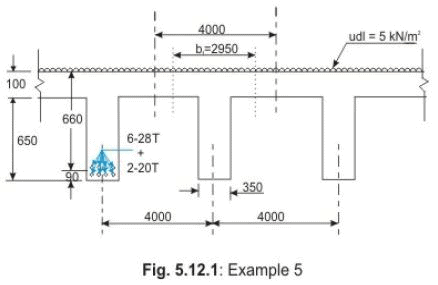Ex.5:  Design the simply supported flanged beam of Fig. 5.12.1, given the following: Df  =  100 mm,  D  =  750 mm,  bw  =  350 mm, spacing of beams  =  4000 mm c/c,  effective span  =  12 m,  cover  =  90 mm,  d  =  660 mm and imposed loads  =  5 kN/m2.  Fe 415 and M 20 are used.

Solution:

Step 1:  Computation of factored bending moment

Weight of slab per m2  =  (0.1) (1) (1) (25)  =  2.5  kN/m2

So, Weight of slab per m    = (4) (2.5) = 10.00 kN/m

Dead loads of web part of the beam  =  (0.35) (0.65) (1) (25)  =  5.6875  kN/m

Imposed loads  =  (4) (5) = 20  kN/m  Total loads  =  30 + 5.6875  =  35.6875  kN/m

Factored Bending moment =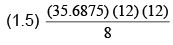963.5625  kNm

Step 2:  Computation of xu,lim

Effective width of flange =(lo/6) + bw+ 6 Df = (12000/6) + 350 + 600 = 2,950 mm.
xu,max  =  0.48 d  =  0.48 (660)  =  316.80 mm. This shows that the neutral axis is in the web of this beam.

D/d   =  100/660  =  0.1515  <  0.2, and

Df /xu   =  100/316.8  =  0.316 <  0.43

The expression of  Mu,lim  is obtained from Eq. 5.7 of Lesson 10 (case ii a of sec. 5.10.4.2) and is as follows:

Mu,lim = 0.36(xu,max /d){1 - 0.42 (xu,max /d)} fck bw d2 + 0.45 fck (bf - bw) Df (d - Df /2)
= 0.36(0.48) {1 - 0.42(0.48)} (20) (350) (650) (650)
+ 0.45 (20) (2950 - 350) (100) (660 - 50)  =  1,835.43 kNm

The design moment  Mu = 963.5625 kNm is less than  Mu,lim. Hence, one underreinforced beam can be designed.

Step 3: Determination of  xu

Since the design moment Mis almost 50% of Mu,lim, let us assume the neutral axis to be in the flange. The area of steel is to be calculated from the moment equation (Eq. 3.23 of Lesson 5), when steel is ensured to reach the design stress fd = 0.87 (415) = 361.05 N/mm2.  It is worth mentioning that the term b of Eq. 3.23 of Lesson 5 is here bf  as the T- beam is treated as a rectangular beam when the neutral axis is in the flange.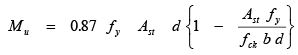(3.23)
Here, all but Ast are known. However, this will give a quadratic equation of Ast and the lower one of the two values will be provided in the beam. The above equation gives: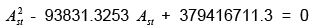which gives the lower value of Ast as: Ast  =  4,234.722097 mm2. The reason of selecting the lower value of Ast is explained in sec 3.6.4.8 of Lesson 6 in the solution of  Design Problem 3.1. Then, employing Eq. 3.16 of Lesson 5, we get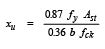(3.16)

or xu  =  71.98 mm. Again, employing Eq. 3.24 of Lesson 5, we can determine xu first and then Ast from Eq. 3.16 or 17 of Lesson 5, as explained in the next step. Eq. 3.24 of Lesson 5 gives:

M =  0.36(xu /d) {1 - 0.42(xu /d)} fck bd2

=  0.36 (xu) {1 - 0.42 (xu /d)} fck bd

963.5625 (106) =  0.36 (xu) {1 - 0.42 (x/660)} (20) (2950) (660)

or xu  =  72.03 mm.

The two values of xu are the same. It is thus seen that, the value of  x can be determined either first finding the value of  Ast, from Eq. 3.23 of Lesson 5 or directly from Eq. 3.24 of Lesson 5 first and then  the value of  Ast can be determined.

Step 4: Determination of  Ast

Equating C = T  , we have from Eq. 3.17 of Lesson 5: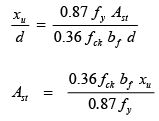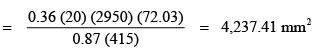Minimum  Ast  =  (0.85/fy) bw d  =  (0.85/415) (350) (660)  =  473.13  mm2

Maximum  Ast  =  0.04 bw D  =  (0.04) (350) (660)  =  9,240  mm2

Hence, Ast  =   4,237.41 mm2 is o.k.

Provide  6 - 28 T (= 3694 mm2) + 2-20 T (= 628 mm2)  to have total  Ast = 4,322 mm2

Ex.6:  Design a beam in place of the beam of Ex.5 (Fig. 5.12.1) if the imposed loads are increased to 12 kN/m2. Other data are: Df = 100 mm, bw = 350 mm, spacing of beams = 4000 mm c/c, effective span = 12 m simply supported and cover = 90 mm. Use Fe 415 and M 20.

Solution:  As in Ex.5,  b= 2,950 mm.

Step 1:  Computation of factored bending moment

Weight of slab/m2  =  2.5 kN/m2 (as in Ex.1)

Imposed loads  =  12.0  kN/m2 (given)

Total weight of slab and imposed loads  =  14.5 (4)  =  58.0  kN/m

Dead loads of the beam  =  0.65 (0.35) (25)  =  5.6875 kN/m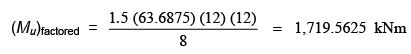Step 2: Determination of  Mu,lim

Mu,lim  of the beam of Ex.5 = 1,835.43 kNm. The factored moment of this problem (1,719.5625 kNm) is close to the value of Mu,lim of the section.

Step 3: Determination of  d

Assuming Df /d    <  0.2, we have from Eq. 5.7 of Lesson 10,

Mu = 0.36(xu,max /d){1 - 0.42 (xu,max /d)} fck bd2 + 0.45 fck (bf - bw) D(d - Df /2)
1719.5625 (106) = 0.36(0.48) {1 - 0.42(0.48)} (20) (350) d2   + 0.45 (2600) (20) (100) (d - 50)

Solving the above equation, we get  d  =  624.09 mm,  giving total depth  =  624.09 + 90 = 715 mm (say).

Since the dead load of the beam is reduced due to decreasing the depth of the beam, the revised loads are calculated below:

Loads from the slab  =  58.0  kN/m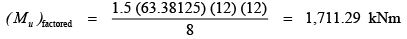Approximate value of  Ast: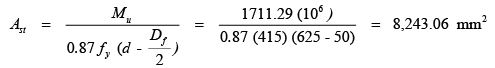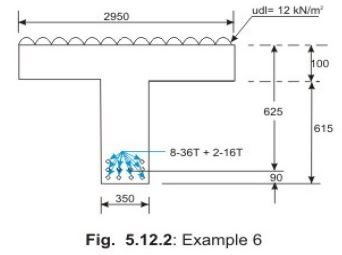Step 4: Determination of Ast (Fig. 5.12.2)

xu  =  xu,max  =  0.48 (625)  =  300 mm

Equating T and C  (Eq. 5.5 of Lesson 10), we have:  0.87 fy Ast   =  0.36 xu,max bw fck + 0.45 fck (bf - bw) Df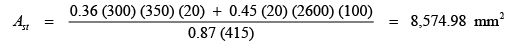Maximum   Ast  =  0.04 b D  =  0.04 (350) (715)  =  10,010.00 mm2

Minimum  Ast  =  (0.85/fy) bw d  =  (0.85/415) (350) (625)  =  448.05 mm2

Hence, Ast  =  8,574.98 mm2    is o.k.

So, provide 8-36 T + 2-18 T  =  8143 + 508  =  8,651 mm2

Step 5: Determination of xu

Using Ast =  8 ,651 mm2 in the expression of  T = C (Eq. 5.5 of Lesson 10), we have:

0.87 fy Ast   =  0.36 xu bw fck +  0.45 fck (bf - bw) Df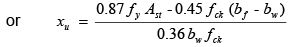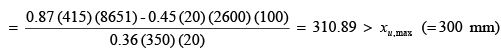So, Ast  provided is reduced to 8-36 + 2-16  =  8143 + 402  =  8,545 mm2.  Accordingly,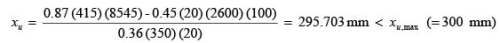Step 6: Checking of  Mu

Df /d  =  100/625  =  0.16  <  0.2 Df /xu  =  100/215.7  =  0.33  <  0.43.

Hence, it is a problem of case (iii a) and Mu can be obtained from Eq. 5.14 of Lesson 10.

So, Mu  =  0.36(xu /d) {1 - 0.42(xu /d)} fck bd2  +  0.45 fck (bf - bw) (Df) (d - Df /2)

= 0.36 (295.703/625) {1 - 0.42 (295.703/625)} (20) (350) (625) (625)
+ 0.45 (20) (2600) (100) (625 - 50)
=  1,718.68  kNm  >  (Mu)design  (= 1,711.29 kNm)

Hence, the design is o.k.

Ex.7:  Determine the tensile reinforcement Ast of the flanged beam of Ex.5 (Fig. 5.12.1) when the imposed loads = 12 kN/m2. All other parameters are the same as those of Ex.5: D= 100 mm, D = 750 mm, bw = 350 mm, spacing of beams = 4000 mm c/c, effective span = 12 m, simply supported, cover = 90 mm and d = 660 mm. Use Fe 415 and M 20.

Solution:

Step 1: Computation of factored bending moment  Mu

Loads/m  = 14.5 (4)  =  58.0  kN/m

Factored Mu  =    (1.5) (63.6875) (12) (12)/8  =  1,719.5625 kNm.

Step 2: Determination of  Mu,lim

From Ex.5, the  Mu,lim  of this beam  =  1,835.43  kNm. Hence, this beam shall be designed as under-reinforced.

Step 3: Determination of  xu

Assuming xu  to   be in the flange, we have from Eq. 3.24 of Lesson 5 and considering b = bf,

Mu  =  0.36x {1 - 0.42(x/d)} fck bf d  1719.5625 (106)

=  0.36 xu {1 - 0.42 (xu /660)} (20) (2950) (550)

Solving, we get  xu  =  134.1  >  100 mm

So, let us assume that the neutral axis is in the web and Df /xu   <  0.43, from Eq. 5.14 of Lesson 10 (case iii a of sec. 5.10.4.3), we have:

M =  0.36(xu /d) {1 - 0.42(xu /d)} fck bw d2  +  0.45 fck (bf - bw) (Df) (d - Df /2)

= 0.36 xu {1 - 0.42 (xu /660)} (20) (350) (660)

+ 0.45 (20) (2600) (100) (660 - 50)
Substituting the value of

Mu  =  1,719.5625  kNm

in the above equation and simplifying, xu - 1571.43 xu + 276042  =  0

Solving, we have

xu  =  201.5 mm Df /xu  =  100/201.5  =  0.496  >  0.43.

So, we have to use Eq. 5.15 and 5.18 of Lesson 10 for yf  and Mu (case iii b of sec. 5.10.4.3).

Thus, we have: Mu  =  0.36 xu {1 - 0.42( xu /d)} fck bd + 0.45  fck (bf - bw) yf (d - yf /2)

where, y=  (0.15 xu + 0.65 Df)

So, Mu  =  0.36 xu {1 - 0.42 (xu/660)} (20) (350) (660)

+ 0.45 (20) (2600) (0.15 x+ 65) (660 - 0.075 xu - 32.5) or 1719.5625 (106 )

=  3.75165 (106) xu - 795.15 xu  +  954.4275 (106)

Solving, we get  xu  =  213.63 mm. Df /xu  =  100/213.63  =  0.468  >  0.43. Hence, o.k.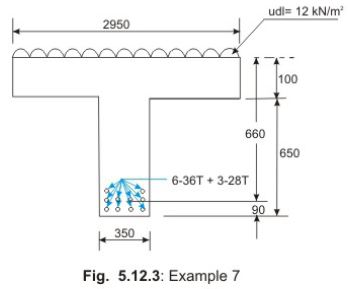Step 4: Determination of  Ast

Equating C = T    from Eqs. 5.16 and 5.17 of Lesson 10 (case iii b of sec. 5.10.4.3), we have:

0.87 fy Ast   =  0.36 fck bw x+ 0.45 fck (bf – bw) yf where, yf =  0.15 x+ 0.65 Df

Here, using   xu  =  213.63 mm,  Df =  100 mm,

we get yf =  0.15 (213.63)  +  0.65 (100)  =  97.04 mm
So,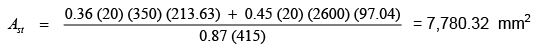Minimum    Ast  =  (0.85/fy) (bw) (d)  =  0.85 (350) (660)/(415)  =  473.13  mm2 Maximum   Ast  =  0.04 bw D  =  0.04 (350) (750)  =  10,500  mm2 Hence,       Ast  =  7,780.32  mm2  is o.k.

Provide  6-36 T + 3-28 T  (6107 + 1847  =  7,954  mm2). Please refer to Fig. 5.12.3.

Step 5: Checking of  x and  Mu  using  Ast  =  7,954  mm2

From  T = C (Eqs. 5.16 and 5.17 of Lesson 10), we have

0.87 fy Ast  =  0.36 fck bxu + 0.45 fck (b– bw) ywhere, yf =  0.15 xu + 0.65 Df

or      0.87 (415) (7954)  =  0.36 (20) (350) xu + 0.45 (20) (2600) (0.15 x+ 0.65 Df)

or xu  =  224.01 mm Df /x =  100/224.01  =  0.446  >  0.43.

Accordingly, employing Eq. 5.18 of Lesson 10 (case iii b of sec. 5.10.4.3), we have:

So, Mu  =  0.36 xu {1 - 0.42( xu /d)} fck bw d + 0.45  fck (b- bw) yf (d - yf /2)

=  0.36 (224.01){1 - 0.42 (224.01/660)} (20) (350) (660)
+ 0.45 (20) (2600) {(0.15) 224.01 + 65} {(660) - 0.15 (112) - 32.5}
=  1,779.439  kNm  >  1,719.5625  kNm

Hence, o.k.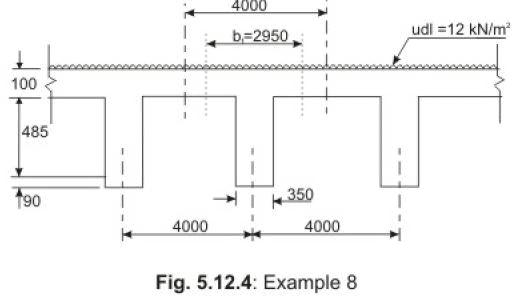Ex.8: Design the flanged beam of Fig. 5.12.4, given in following:  Df = 100 mm, D = 675 mm, b= 350 mm, spacing of beams = 4000 mm c/c, effective span = 12 m simply supported, cover = 90 mm, d = 585 mm and imposed loads = 12 kN/m2.  Use Fe 415 and M 20.

Step 1: Computation of factored bending moment, M

Weight of slab/m2  =  (0.1) (25)  =  2.5  kN/m2

Total weight of slab + imposed loads/m  =  14.5 (4)  =  58  kN/m

Factored Mu  =    (1.5) (63.032) (12) (12)/8  =  1,701.87  kNm

Step 2: Determination of  Mu,lim

Assuming the neutral axis to be in the web, Df /xu <  0.43 and Df /d = 100 / 585 = 0.17  < 0.2, we consider the case (ii a) of sec. 5.10.4.2 of Lesson 10 to get the following:

Mu,lim  =  0.36 (xu,max /d) {1 – 0.42 (xu,max /d)} fck bw d2

+ 0.45 fck (b– bw) Df (d – Df /2)

=  0.36(0.48) {1 – 0.42 (0.48)} (20) (350) (585) (585)
+  0.45(20) (2600) (100) (585 – 50) =  1,582.4  kNm

Since, factored  Mu  >  Mu,lim, the beam is designed as doubly reinforced.

Mu2  =  Mu - Mu,lim  =  1701.87 - 1582.4  =  119.47 kNm

Step 3: Determination of area of steel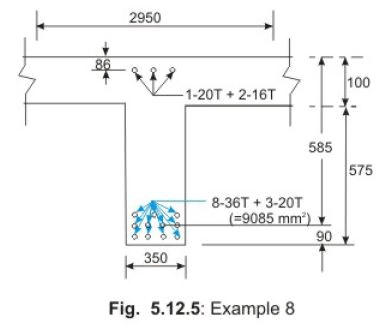Ast,lim  is obtained equating  T = C  (Eqs. 5.5 and 6 of Lesson 10).

0.87 fy (Ast, lim)  =  0.36 bw (xu,max /d) d fck  +  0.45 fck (bf - bw) D
or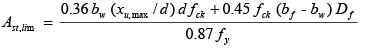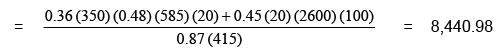mm2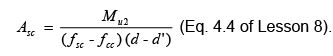where   fsc     =  353  N/mm2  for  d'/d  =  0.1 fcc

=  0.446 fck  =  0.446 (20)  =  8.92  N/mm

Mu2   =  119.47 (106)  Nmm

d'     =  58.5  mm

d     =  585  mm

Using the above values in the expression of  Asc (Eq. 4.4 of Lesson 8), we get Asc  =  659.63  mm2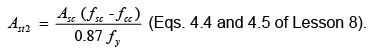Substituting the values of  Asc,  fsc,  fcc  and  f we get Ast =  628.48  mm2

Total Ast  = Ast,lim  + Ast2  =  8,440.98 + 628.48  =  9,069.46  mm2

Maximum Ast  =  0.04 bw D  =  0.04 (350) (675)  =  9,450 mm2and minimum  Ast  =  (0.85/fy) bw d  =  (0.85/415) (350) (585)  =  419.37 mm2

Hence,   Ast  =  9, 069.46 mm2 is o.k.

Provide  8-36 T + 3-20 T  =  8143 + 942  =  9,085  mm for  Ast and 1-20 + 2-16 = 314 + 402 = 716 mm2  for  Asc  (Fig. 5.12.5).

Step 4: To check for  xu  and  Mu  (Fig. 5.12.5)

Assuming  xu  in the web and  D/x <  0.43  and using  T = C (case ii a of sec. 5.10.4.2 of Lesson 10 with additional compression force due to compression steel), we have:

0.87 fy Ast  =  0.36 bw xu  fck  +  0.45 (b- bw) fck D+ Asc (fsc - fcc)

or 0.87 (415 ) (908 5)  =  0.36 (350) xu (20) + 0.45 (2600) (20) (100)

+ 716 {353 - 0.45 (20)}

This gives  xu  =  275.33 mm. xu,max  =  0.48 (d)  =  0.48 (585)  =  280.8 mm.

So, xu  <  xu,max,  D/x =  100/275.33  =  0.363  <  0.43   and   D/d =  100/585  =  0.17  <  0.2.

The assumptions, therefore, are correct. So, Mu  can be obtained from Eq. 5.14 of sec. 5.10.4.3 of Lesson 10 with additional moment due to compression steel, as given below:
So, Mu  =  0.36 bw xu fck (d - 0.42 xu) + 0.45 (bf - bw) fck D(d - Df /2)

+  Asc (fsc - fcc) (d - d')

=  0.36 (350) (275.33) (20) {585 - 0.42 (275.33)}
+ 0.45 (2600) (20) (100) (585 - 50) + 716 (344) (585 - 58.5)
=  325.66 + 1251.9 + 129.67  =  1,707.23  kNm

Factored moment  =  1,701.87 kNm < 1,707.23 kNm.  Hence, o.k.

5.12.4 Practice Questions and Problems with Answers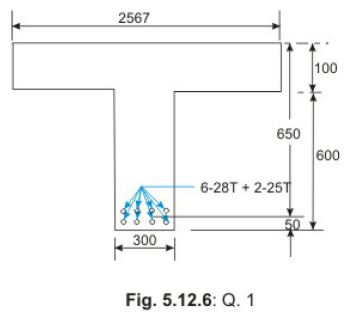Q.1:  Determine the steel reinforcement of a simply supported flanged beam (Fig. 5.12.6) of  Df = 100 mm, D  =  700 mm, cover = 50 mm,  d  =  650 mm,  bw  =  300 mm, spacing of the beams = 4,000 mm c/c, effective span = 10 m and imposed loads = 10 kN/m2.  Use M 20 and Fe 415.
A.1: Solution:

Step 1:  Computation of (Mu)factored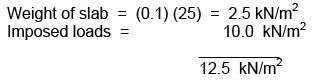The design moment  M= 963.5625 kNm is less than  Mu,lim.

Hence, one underreinforced beam can be designed.

Total loads per m = (12.5) (4) = 50 kN/m

Factored Mu =  ( 1.5) (54.50) (10) (10)/8  =  1,021.87 kNm

Step 2:  Determination of  Mu,lim

Effective width of the flange b= lo/6 + bw + 6 Df = (10,000/6) + 300 + 600 = 2,567 mm.

xu,max  =  0.48 d  =  0.48 (650)  =  312 mm

Hence, the balanced neutral axis is in the web of the beam.

D/d  =  100/650  =  0.154  <  0.2 Df /xu  =  100/312  =  0.32  <  0.43

So, the full depth of flange is having a stress of 0.446 fck.

From Eq. 5.7 of Lesson 10 (case ii a of sec. 5.10.4.2), we have,

Mu,lim  =  0.36 (xu,max /d) {1 – 0.42 (xu,max /d)} fck bw d   + 0.45 fck (b– bw) Df (d – Df /2)

=  0.36(0.48) {1 – 0.42(0.48)} (20) (300) (650) (650)
+  0.45(20) (2267) (100) (650 – 50)
=  1573.92 kNm  >  Mu (= 1021.87 kNm) So, the beam will be under-reinforced one.

Step 3:  Determination of  xu

Assuming xu is  in the flange, we have from Eq. 3.24 of Lesson 5 (rectangular beam when b = bf ).

Mu  =  0.36 (x/d) {1 – 0.42 (xu /d)} fck bf d2

=  0.36 xu {1 – 0.42 (xu /d)} fck bd 1021.87 (106)

=  0.36 xu {1 – 0.42(xu/650)} (20) (2567) (650)

xu  =  89.55  mm2  <  100 mm (Hence, the neutral axis is in the flange.)

Step 4:  Determination of  Ast

Equating C = T  , we have from Eq. 3.17 of Lesson 5: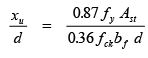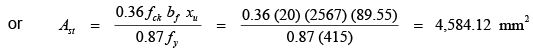Minimum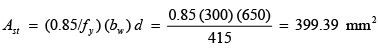Maximum  Ast  =  0.04 bD  =  (0.04) (300) (700)  =  8,400  mm2

So,     Ast  =  4,584.12  mm2  is o.k.

Provide    6-28 T + 2-25 T  =  3694 + 981  =  4,675  mm2  (Fig. 5.12.6

5.12.6 Test 12 with Solutions

Maximum Marks = 50,
Maximum Time = 30 minutes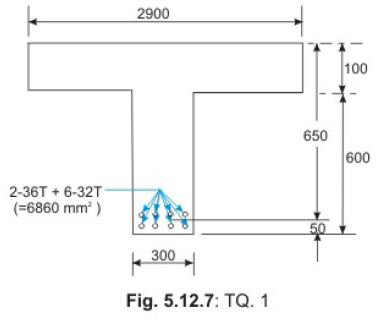TQ.1: Determine the steel reinforcement Ast of the simply supported flanged beam of
Q.1
(Fig. 5.12.6) having Df = 100 mm, D = 700 mm, cover = 50 mm,  d  =  650 mm,  bw  =  300 mm, spacing of the beams = 4,000 mm c/c, effective span = 12 m and imposed loads = 10 kN/m2. Use M 20 and Fe 415.
A.TQ.1:

Solution: Step 1:  Computation of (Mu)factored

Total loads from Q.1 of sec. 5.12.4 = 54.50  kN/m

Factored M =    (1.5) (54.50) (12) (12)/8  =  1,471.5  kNm

Step 2:  Determination of  Mu,lim

Effective width of flange  =  lo/6 + b+ 6

D= (12000/6) + 300 + 600  =  2,900 mm  (Fig. 5.12.7)

xu,max  =  0.48 d  =  0.48 (650)  =  312 mm

Hence, the balanced neutral axis is in the web.

Df /d  =  100/650  =  0.154 < 0.2

Df /xu  =  100/312  =  0.32 < 0.43

So, the full depth of flange is having constant stress of 0.446 fck. From Eq. 5.7 of Lesson 10 (case ii a of sec. 5.10.4.2), we have

Mu,lim  =  0.36 (xu,max /d) {1 – 0.42 (xu,max /d)} fck bd   + 0.45 fck (b– bw) Df (d – Df /2)

=  0.36(0.48) {1 – 0.42(0.48)} (20) (300) (650) (650) +  0.45(20) (2600) (100) (650 – 50) =  1,753.74  kNm  >  1,471.5  kNm

So, the beam will be under-reinforced.

Step 3:  Determination of  x

Assuming xu to b e in the flange, we have from Eq. 3.24 of Lesson 5 (singly reinforced rectangular beam when b =  bf ):

M =  0.36 xu {1 – 0.42 (xu /d)} fck bf d

or 1471.5 (106 )  =  0.36 (xu) {1 – 0.42 (xu /650)} (20) (2900) (650)

or xu2 – 1547.49 x+ 167.81 (103)  =  0

Solving, we have  xu  =  117.34 mm > 100 mm

So, neutral axis is in the web. Assuming  Df /xu < 0.43, we have from Eq. 5.14 of Lesson 10 (case iii a of sec. 5.10.4.3),

Mu  =  0.36 xu {1 – 0.42 (xu /d)} fck bw d  + 0.45 fck (b– bw) Df (d – Df /2)

=  0.36 xu {1 – 0.42 (xu/650)} (20) (300) (650)   +  0.45(20) (2600) (100) (650 – 50)

or xu– 1547.62  x+ 74404.7  =  0

Solving, we have  xu  =  49.67  <  100 mm

However, in the above when it is assumed that the neutral axis is in the flange xu is found to be 117.34 mm and in the second trial when xu is assumed in the web  xu is seen to be 49.67 mm. This indicates that the full depth of the flange will not have the strain of 0.002, neutral axis is in the web and  Df /xu is more than 0.43. So, we have to use Eq. 5.18 of Lesson 10, with the introduction of  yf  from Eq. 5.15 of Lesson 10.

Assuming Df /xu  > 0.43, from Eqs. 5.15 and 5.18 of Lesson 10 (case iii b of sec. 5.10.4.3), we have:

Mu  =  0.36 xu {1 – 0.42 (xu /d)} fck bd  + 0.45 fck (b– bw) yf (d – yf /2)

where, y = (0.15 xu + 0.65 Df) So,

Mu =  0.36 xu {1 – 0.42 (xu/650)} (20) (300) (650)   +  0.45(20) (2600) (0.15 xu + 0.65) (650 – 0.075 xu – 0.325 xu)

or, 1471.5 (10 6)  =  - 1170.45 xu2 + 3.45735 xu + 939.2175 (106)

Solving, we get  xu  =  162.9454  mm.

This shows that the assumption of  Df /xu > 0.43 is correct as  Df /xu  = 100 / 162.9454 = 0.614.

Step 4:  Determination of  Ast

Equating C = T    from Eqs. 5.16 and 5.17 of Lesson 10 (case iii b of sec. 5.10.4.3), we have

0.87 fy Ast   =  0.36 fck bw x+ 0.45 fck (b– bw) yf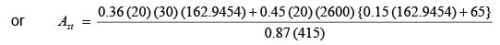=  974.829 + 5,796.81  =  6,771.639  mm2Minimum

Ast  =  (0.85/fy) (bw) (d)  =  0.85 (300) (650)/415  =  399.39  mm2

Maximum   Ast  =  0.04 (bw) (D)  =  0.04 (300) (700)  =  8,400  mm2

So,       Ast  =  6,771.639  is o.k. Provide  2-36 T + 6-32 T  =  2035 + 4825  =  6,860  mm >  6,771.639  mm2 (Fig. 5.12.7).

Summary of this Lesson

This lesson explains the steps involved in solving the design type of numerical problems. Further, several examples of design type of numerical problems are illustrated explaining the steps of their solutions. Solutions of practice problems and test problems will give the readers confidence in applying the theory explained in Lesson 10 in solving the numerical problems.

Offer running on EduRev: Apply code STAYHOME200 to get INR 200 off on our premium plan EduRev Infinity!

## RCC & Prestressed Concrete

14 videos|24 docs|12 tests

,

,

,

,

,

,

,

,

,

,

,

,

,

,

,

,

,

,

,

,

,

;